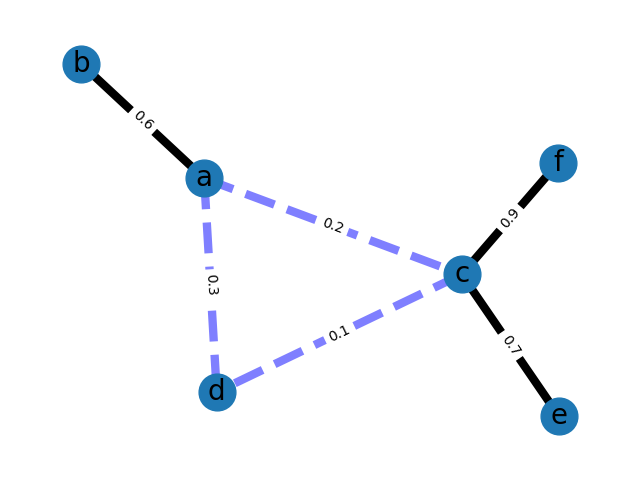# Weighted Graph#

An example using Graph as a weighted network.```import matplotlib.pyplot as plt
import networkx as nx

G = nx.Graph()

elarge = [(u, v) for (u, v, d) in G.edges(data=True) if d["weight"] > 0.5]
esmall = [(u, v) for (u, v, d) in G.edges(data=True) if d["weight"] <= 0.5]

pos = nx.spring_layout(G, seed=7)  # positions for all nodes - seed for reproducibility

# nodes
nx.draw_networkx_nodes(G, pos, node_size=700)

# edges
nx.draw_networkx_edges(G, pos, edgelist=elarge, width=6)
nx.draw_networkx_edges(
G, pos, edgelist=esmall, width=6, alpha=0.5, edge_color="b", style="dashed"
)

# node labels
nx.draw_networkx_labels(G, pos, font_size=20, font_family="sans-serif")
# edge weight labels
edge_labels = nx.get_edge_attributes(G, "weight")
nx.draw_networkx_edge_labels(G, pos, edge_labels)

ax = plt.gca()
ax.margins(0.08)
plt.axis("off")
plt.tight_layout()
plt.show()
```

Total running time of the script: ( 0 minutes 0.096 seconds)

Gallery generated by Sphinx-Gallery